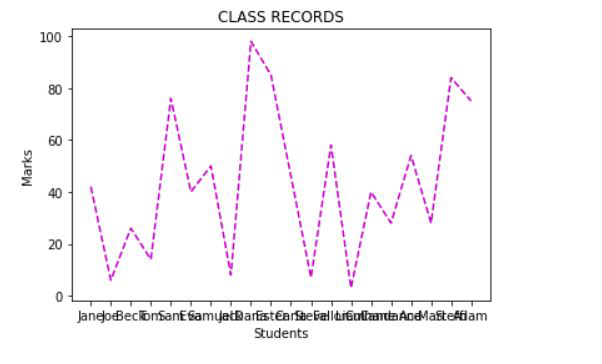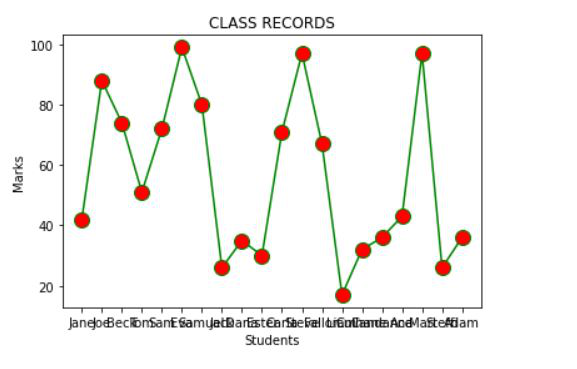Related Articles

# Line plot styles in Matplotlib

• Last Updated : 11 Dec, 2020

Python is a high-level, interpreted, and dynamically typed programming language that can be used to manage huge datasets. Python supports a wide variety of data visualization libraries like Matplotlib, Seaborn, Bokeh, Geoplotlib, Ggplot, and Plotly. Among all these libraries, Matplotlib is comparatively simple and easy to implement. The Matplotlib library of Python is a popular choice for data visualization due to its wide variety of chart types and its properties that can be manipulated to create chart styles. The matplotlib.pyplot.plot(*args, **kwargs) method of matplotlib.pyplot is used to plot the graph and specify the graph style like color or line style.

The following line styles are available in Matplotlib:

Color code abbreviations that can be used along with the line styles:

The following examples demonstrate plotting graphs having different line styles:

Example 1:

In this example, the matplotlib.pyplot library is imported. The student names are added to the student’s list and the marks list is created at the random.randint() method. Next, the X and Y axis are labeled and the graph is given a title. Finally, the graph is plotted using the plot() method of matplotlib.pyplot. Here the abbreviated form of color and line style is used. The color abbreviation chosen is ‘m’ which is magenta and the line style chosen is ‘–‘ which is dashed line style.

Below is the implementation:

## Python3

 `import` `matplotlib.pyplot as plt ` `import` `random as random ` ` `  `students ``=` `[``"Jane"``,``"Joe"``,``"Beck"``,``"Tom"``, ` `            ``"Sam"``,``"Eva"``,``"Samuel"``,``"Jack"``, ` `            ``"Dana"``,``"Ester"``,``"Carla"``,``"Steve"``, ` `            ``"Fallon"``,``"Liam"``,``"Culhane"``,``"Candance"``, ` `            ``"Ana"``,``"Mari"``,``"Steffi"``,``"Adam"``] ` ` `  `marks``=``[] ` `for` `i ``in` `range``(``0``,``len``(students)): ` `     ``marks.append(random.randint(``0``, ``101``)) ` ` `  `plt.xlabel(``"Students"``) ` `plt.ylabel(``"Marks"``) ` `plt.title(``"CLASS RECORDS"``) ` `plt.plot(students,marks,``'m--'``) `

Output:Example 2:

In this example, the matplotlib.pyplot library is imported. The student names are added to the student’s list and the marks list is created by random.randint() method. Next, the X and Y axis are labeled and the graph is given a title. Finally, the graph is plotted using the plot() method of matplotlib.pyplot. Here instead of using the abbreviated format, the line properties are controlled using keyword arguments. The keywords are assigned required values. The color used is green, the linestyle is solid, the marker is circle marker, the marker color is red and the marker size is 12px.

Below is the implementation:

## Python3

 `import` `matplotlib.pyplot as plt ` `import` `random as random ` ` `  `students ``=` `[``"Jane"``,``"Joe"``,``"Beck"``,``"Tom"``,``"Sam"``, ` `            ``"Eva"``,``"Samuel"``,``"Jack"``,``"Dana"``,``"Ester"``, ` `            ``"Carla"``,``"Steve"``,``"Fallon"``,``"Liam"``,``"Culhane"``, ` `            ``"Candance"``,``"Ana"``,``"Mari"``,``"Steffi"``,``"Adam"``] ` ` `  `marks``=``[] ` `for` `i ``in` `range``(``0``,``len``(students)): ` `     ``marks.append(random.randint(``0``, ``101``)) ` ` `  ` `  `plt.xlabel(``"Students"``) ` `plt.ylabel(``"Marks"``) ` `plt.title(``"CLASS RECORDS"``) ` `plt.plot(students, marks, color ``=` `'green'``, ` `         ``linestyle ``=` `'solid'``, marker ``=` `'o'``, ` `         ``markerfacecolor ``=` `'red'``, markersize ``=` `12``) `

Output:Attention geek! Strengthen your foundations with the Python Programming Foundation Course and learn the basics.

To begin with, your interview preparations Enhance your Data Structures concepts with the Python DS Course. And to begin with your Machine Learning Journey, join the Machine Learning – Basic Level Course

My Personal Notes arrow_drop_up
Recommended Articles
Page :